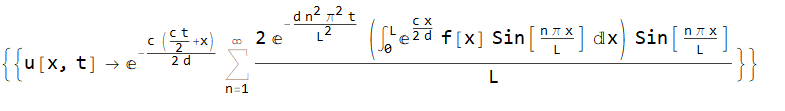## Question:How do I plot this function with the plot...

i want to plot

u(x,y,z,t)=tanh(x+y+z-wt),  (w is a constant)

## how to do reversible hash on 2d array ?...

i would like to to test and do hash on a 2d array and then predict this hash and then reverse back to another hash which can convert back to 2d array , how to do?

## evaluate difficult function...

Dear Community,

I would like to evaluate this difficult function:Unfortunately when I try to define it in Maple, I always get an error message. I tried it in many different ways, but always failed. See also the attached workbook. Can someone pls. have a look, what I do wrong?

best regards

Andras

DifficultFunction.mw

## What happen? sqrt()= same result...

Hi guys, Maple always shows the same result when I use sqrt() functionHow can I fix it? Thanks

## Win10 high contrast mode...

I'm using Win10, maple 2016.

I'm using high contrast mode (dark mode, white on black). Maple looks broken, the text is black on black, and in the side panel, some of the buttons are shiney white.

How do I set maple to support high contrast?

## How to restrict the domain of a variable when find...

Consider two polynomials p and q in t. For example,and. I want to find. But before I find it, solve an inequality and obtain that. After that when I evaluate, I dont want maple to returnas Gcd becauseand thus the factoris an unwanted common divisor. Is there a way to tell maple that "find the Gcd over a spesific domain". Because, otherwise maple needlessly spend time for finding the gcd. Any help would be appreciated.

## some issues with doing integral ...

Hi guys,

I have problem with doing a complicated integral !

cheers.

Question.mw

## How to write the sequence...

This is a simple model of the covid infection as it applies here, down under, without the use of any de's like

https://www.mapleprimes.com/posts/212100-Exploring-The-CoVid19-Outbreak

I'm told a substantial number of people do recover within 45 days ....
So on the 45th day 1 person has recovered, on day 50 two people have recovered and so on.

Just want to know how to write this sequence...

Covid_dbling.mw

## How do I solve an unknown that makes a bivariate p...

I have a bivariate polynomial in x,y,say P, and a parameter, say d, appears in P. I want to find d for which P has at least one non trivial factor. Is there  a fats way to do this in maple. Any help would be appreciated.

## Changing terms to be a function...

Hi,

I am struggling to find a way to change a term like x to x(t) automatically. If I have something like

```Data := x^2

diff(Data, x);
```

the result is 2x

How can I change this 2x to be 2x(t)?

Thanks,

Baharm31

## Solving simultaneous ODEs...

Hi guys,

I am trying to find x(t) from these two equations:

```odeA := m*diff(x(t), t, t) = -m*A*sin(2*Pi*f*t) - k*x(t) + 0.5*q(t)*(N__f*epsilon__0*L*(-2*x(t)/(G^2 - x(t)^2) + 2*(G__1^2 - x(t)^2)*x(t)/(G^2 - x(t)^2)^2)*(G^2 - x(t)^2)/(2*tan(alpha)*(G__1^2 - x(t)^2)) + N__f*epsilon__0*L*(-2*x(t)/(G^2 - x(t)^2) + 2*(G__2^2 - x(t)^2)*x(t)/(G^2 - x(t)^2)^2)*(G^2 - x(t)^2)/(2*tan(alpha)*(G__2^2 - x(t)^2)))/(N__f*epsilon__0*L*ln((G__1^2 - x(t)^2)/(G^2 - x(t)^2))/(2*tan(alpha)) + N__f*epsilon__0*L*ln((G__2^2 - x(t)^2)/(G^2 - x(t)^2))/(2*tan(alpha)) + C__p) - d*diff(x(t), t);

diff(q(t), t) = (q(t)/(N__f*epsilon__0*L*ln((G__1^2 - x(t)^2)/(G^2 - x(t)^2))/(2*tan(alpha)) + N__f*epsilon__0*L*ln((G__2^2 - x(t)^2)/(G^2 - x(t)^2))/(2*tan(alpha)) + C__p) + V__bias)/R1

```

How can I use dsolve to find x(t), apart from q(t) all other parameters are constant.

Thanks,

Baharm31

## Why Maple pdsolve still unable to solve 1D convect...

Can one hope that next version of Maple will be able to solve the standard convection-diffusion pde in 1D?

`pde:=diff(u(x,t),t)=d*diff(u(x,t),x\$2)+c*diff(u(x,t),x);`

in 1D. Even the most simple form with zero boundary conditions can't be solved.

```pde:=diff(u(x,t),t)=d*diff(u(x,t),x\$2)+c*diff(u(x,t),x);
bc:=u(0,t)=0,u(L,t)=0;
ic:=u(x,0)=f(x);
sol:=pdsolve([pde,ic,bc],u(x,t)) assuming d>0,c>0,L>0

sol := NULL```

I tried some HINT's also but not all of them.

FYI, Mathematica 12.1 is now able to solve the above PDE

```ClearAll["Global`*"];
pde=D[u[x,t],t]==d*D[u[x,t],{x,2}]+c*D[u[x,t],x];
bc={u[0,t]==0,u[L,t]==0};
ic=u[x,0]==f[x];
DSolve[{pde,bc,ic},u[x,t],{x,t},Assumptions->{d>0,c>0,L>0}]```

GivesIs it possible I am overlooking some other option or a trick to obtain solution for the above using pdsolve? Only reason I am asking is that Maple can solve much more complicated PDE's and the above is one of the basic diffusion based PDE's. So I am surprised why Maple still can't solve this as it is just a seperation of variables method.

Maple 2020 on windows 10. Physics version 631

## implicit plot of function...

Why is my figure not smooth? How can I make it smooth?## Whose minimum value is more reliable？...

I have a problem about extremal function.

The function is shown below with restricted condition of x and a:  x>=2 and -1 < a <0.

(x-3/2)^a-(x-1)^a+(1-2^(a-1))*((x-3/2)^a-(x-1/2)^a)-x*((x-3/2)^a+x^a-(x-1/2)^a-(x-1)^a)

I guess that  it's minimum value is greater than 0.  In other words,  I want prove that  (x-3/2)^a-(x-1)^a+(1-2^(a-1))*((x-3/2)^a-(x-1/2)^a)-x*((x-3/2)^a+x^a-(x-1/2)^a-(x-1)^a)>0

when I use Minimize function,

Optimization:-Minimize((x-3/2)^a-(x-1)^a+(1-2^(a-1))*((x-3/2)^a-(x-1/2)^a)-x*((x-3/2)^a+x^a-(x-1/2)^a-(x-1)^a),{x>=2, -1<a, a<0})

I 'm not seeing but Error, (in Optimization:-NLPSolve) strict inequalities are not valid constraints. I cannot but add the condition of equality of a : -1<=a, a<=0.

Optimization:-Minimize((x-3/2)^a-(x-1)^a+(1-2^(a-1))*((x-3/2)^a-(x-1/2)^a)-x*((x-3/2)^a+x^a-(x-1/2)^a-(x-1)^a),{x>=2, -1<=a, a<=0})

A result is returned with a warning:
Warning, no iterations performed as initial point satisfies first-order conditions
[0.000000, [a = -2.225074*10^(-308), x = 2.000000]]

Local or Global ?

When I read recent question post

https://www.mapleprimes.com/questions/229132-Maximize-Function-Does-Not-Work?ref=Feed:MaplePrimes:New%20Questions

I'm worrying  that Maple only returns local  extremun. So I use the freely  maple package DirectSearch. We can get it from  https://www.maplesoft.com/applications/view.aspx?SID=101333.

DirectSearch:-GlobalOptima(-((x-3/2)^a-(x-1)^a+(1-2^(a-1))*((x-3/2)^a-(x-1/2)^a)-x*((x-3/2)^a+x^a-(x-1/2)^a-(x-1)^a)),{x>=2, -1<a, a<0},maximize);

Note: I add a  minus sign to find maximize value .

It returns: [0.002264, [a = -0.000203, x = 1.019590*10^13], 605]

But I substitute the value of a and x  into the function，it returns strange result:   4078.360000 not  -0.002264.

I’m confused..

I try to use  Mathematica,

NMinimize[{(x - 3/2)^a - (x - 1)^
a + (1 - 2^(a - 1))*((x - 3/2)^a - (x - 1/2)^a) -
x*((x - 3/2)^a + x^a - (x - 1/2)^a - (x - 1)^a),
x >= 2 && a > -1 && a <= 0}, {x, a}]

It retruns {0., {x -> 13.256, a -> 0.}}  May be well.

mimimize.mw

﻿